Function Repository Resource:

# Orthocenter

Return the orthocenter of a triangle

Contributed by: Ed Pegg Jr
 ResourceFunction["Orthocenter"][{p1,p2,p3}] returns the orthocenter of the triangle defined by vertices p1,p2 and p3.

## Details

The orthocenter is the point where all altitudes of a triangle intersect.
ResourceFunction["Orthocenter"][Triangle[{p1,p2,p3}]] is equivalent to ResourceFunction["Orthocenter"][{p1,p2,p3}].

## Examples

### Basic Examples (2)

Find the orthocenter of three triangle vertices:

 In:=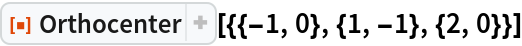Out=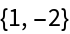Show the triangle with its orthocenter and altitudes:

 In:=Out=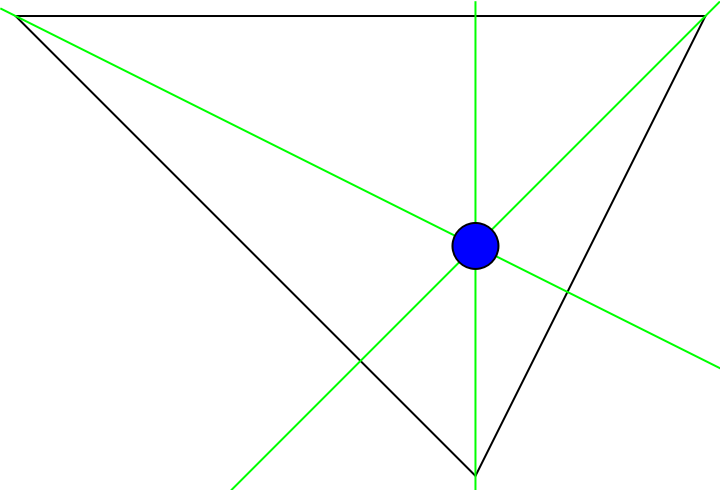### Scope (2)

Compute the orthocenter of a 3D triangle:

 In:=Out=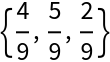Show the orthocenter and the triangle together:

 In:=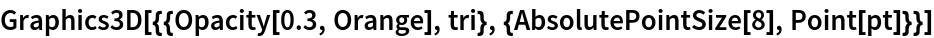Out=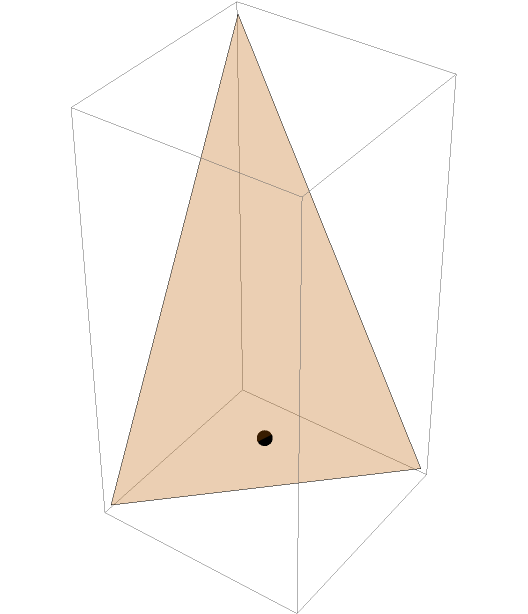### Neat Examples (1)

When four given points comprise a triangle and its orthocenter, and any three of the points are selected as triangle vertices, the remaining point will be the triangle's orthocenter:

 In:=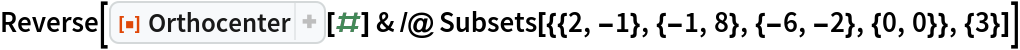Out=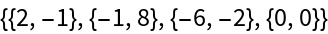In:=Out=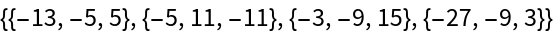## Version History

• 1.1.0 – 24 August 2021
• 1.0.0 – 16 October 2019